MrDenniz

•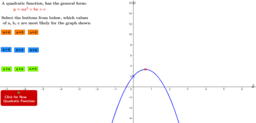Coefficients of a general quadratic function

Activity

MrDenniz

•Circle Theorem 3 - Angles in the Same Segment

Activity

MrDenniz

•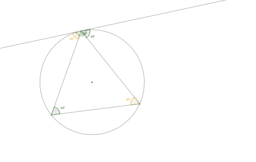Circle Theorem 8 - Alternate Segment Theorem

Activity

MrDenniz

•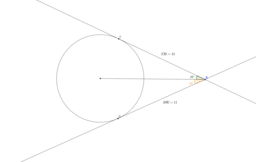Circle Theorem 7 - Tangents from a Point to a Circle II

Activity

MrDenniz

•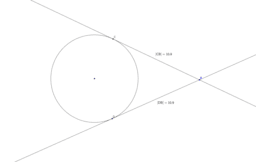Circle Theorem 6 - Tangents from a Point to a Circle

Activity

MrDenniz

•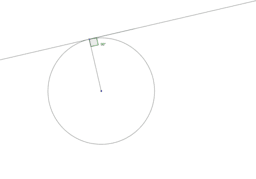Circle Theorem 5 - Radius to a Tangent

Activity

MrDenniz

•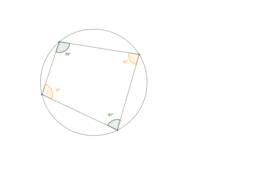Circle Theorem 4 - Cyclic Quadrilateral

Activity

MrDenniz

•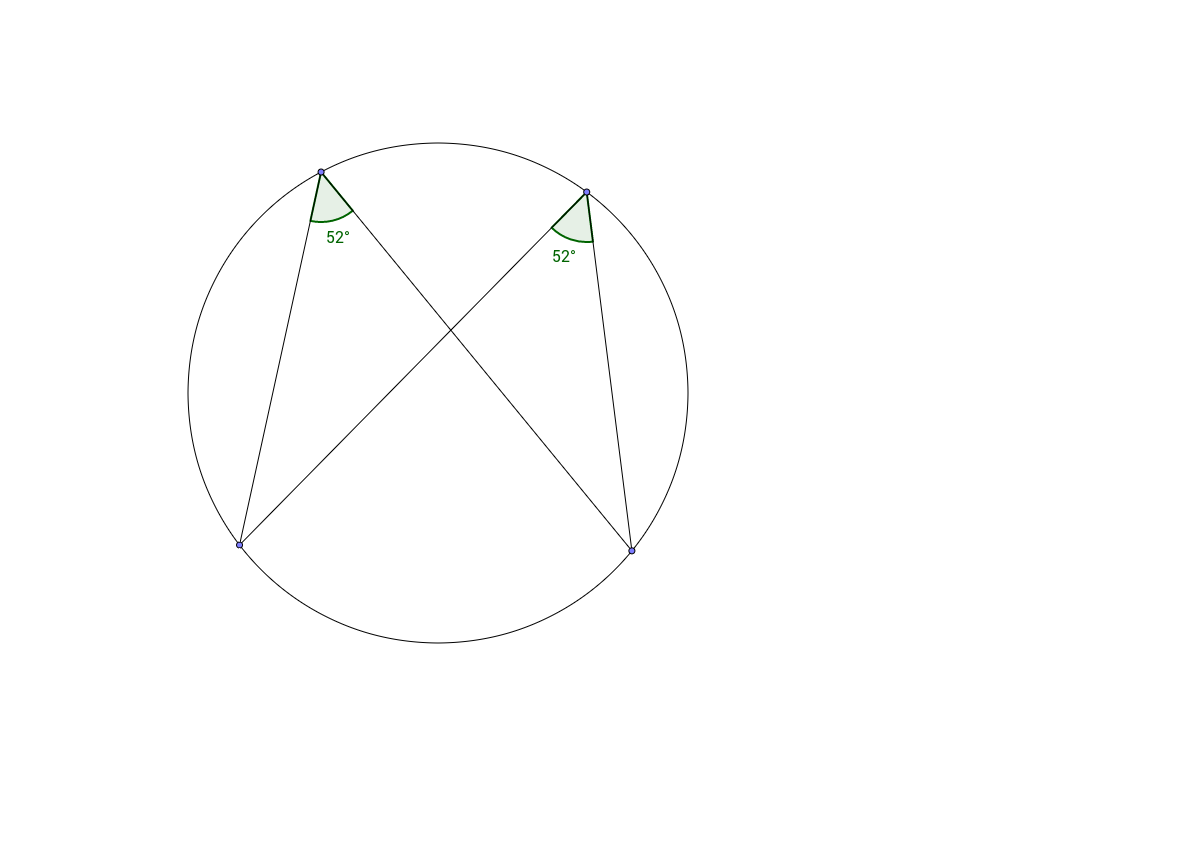Circle Theorem 3 - Angles in the Same Segment

Activity

MrDenniz

•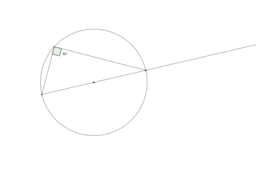Circle Theorem 2 - Angles in a Semicircle

Activity

MrDenniz

•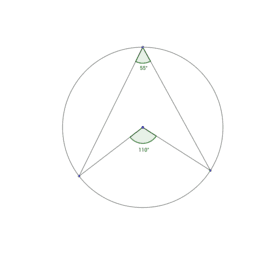Circle Theorems

Book

MrDenniz

•Circle Theorem 1 - Angle at the Centre

Activity

MrDenniz

•Theorem 1

Activity

MrDenniz

•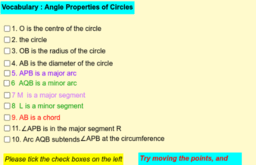CIRCLE THEOREM

Book

MrDenniz

•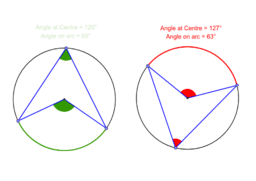Circle Theorems

Book

MrDenniz

•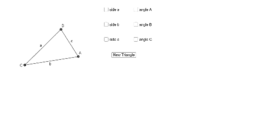Law of Sines, Law of Cosines Self-Check

Activity

MrDenniz

•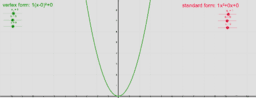Copy of Compare Quadratic Standard Form and Vertex Form

Activity

MrDenniz

•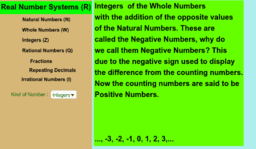The Real Number System

Activity

MrDenniz

•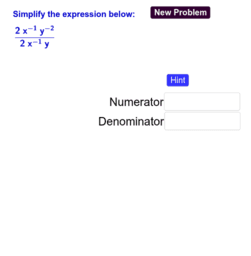Quotient Property of Exponents

Activity

MrDenniz

•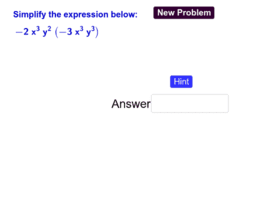Product Property of Exponents

Activity

MrDenniz

•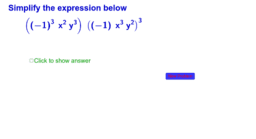Copy of Simplify Expressions with Exponents-Section 4.2

Activity

MrDenniz

•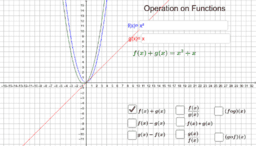Operation on Functions

Activity

MrDenniz

•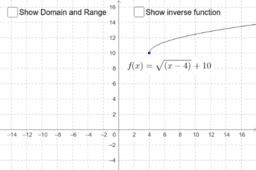Copy of Domain and Range for a square-root function

Activity

MrDenniz

•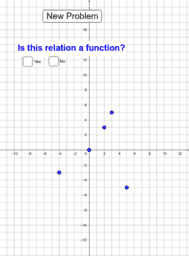Is the relation a function?

Activity

MrDenniz

•